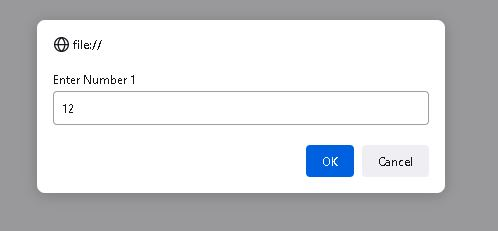In this Javascript program we will learn how to add two integers and return the out put of those two numbers. To add any numbers in Javascript  we will use '+' addition operator.

In this example we will write different ways to add two numbers in Javascript

Example 1: Define Two numbers and add them



Output:

The addition of numbers 21 and 72 is 93

In the above example we define numbers and add them using '+' operator. Now let's add numbers take user inputs.

Example 2: Addition of two numbers from user inputs

In this example we will prompt user to enter numbers which needs to add. To prompt the user we will use prompt () method.



Javascript Addition of Two numbers using user inputs

In the above program we take inputs from user and parse them into Integer then adding them.Output:

The addition of numbers 12 and 22 is 34